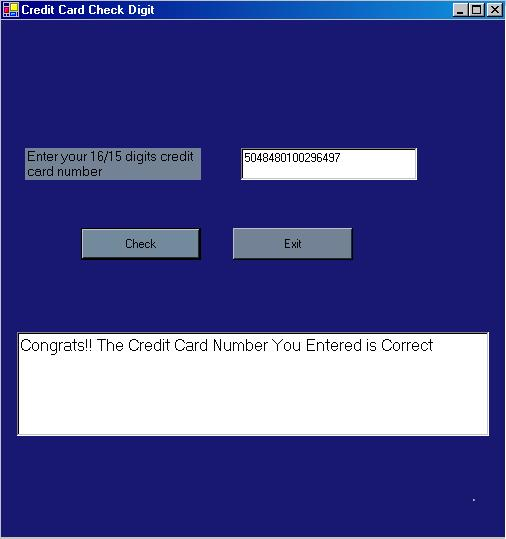# Low Cost Credit Card Verification

Description:

The last digit of a credit card is a check digit that is used to detect keystroke errors. when a check card is entered into a computer. In this article I implemented a check digit algorithm which is public(ISO 2894),which is not widely Known. This check digit Algorithm is designed to catch transposed digits or other typing errors. It is designed as a general-purpose security mechanism. Unfortunately, some businesses have used the algorithm as allow-cost way of "verifying" credit numbers. For example, some online services have deployed software that creates accounts for any individual presenting a credit card number that passes the algorithm, in an attempt to save the cost of performing millions of verifications.

The algorithm is:

1. Multiply each digit in the credit card by its "weight". If a charge card has an even number(16 digit card number) of digits, the first digit has a weight of 2, otherwise the digit has weight of 1.
Afterwards, the weights of the digits alternate 1,2,1,2.
2. If any digit has a weighted value that is more than 9, Subtract 9.
3. Add together the weights of all the digits, modulo 10.
4. the result should be zero for a valid credit card.

Examples:

1. Here is the card number: 3728 024906 54059
The above charge card has 15 digits. the number is odd, So the first digit has a weight of 1.

to compute the check digit, we multiply :

(3*1),(7*2),(2*1),(8*2),(0*1),(2*2),(4*1),(9*2),(0*1),(6*2),(5*1),(4*2),(0*1),(5*2),(9*1)
which is :

3 14 2 16 0 4 4 18 5 0 12 5 8 0 10 9

Substract 9 from every value greater than 9, and add them together:
3+5+2+7+0+4+4+9+0+3+5+8+0+1+9 = 60
This gives a check of 0, because 60 mod 10 = 0;
Hence the Credit card is Valid.

2. Here is another card number : 5048 4801 0029 6497
The above charge card has 16 digits. the number is odd, So the first digit has a weight of 2.
to compute the check digit, we multiply :

(5*2),(0*1),(4*2),(8*1),(4*2),(8*1),(0*2),(1*1),(0*2),(0*1),(2*2),(9*1),(6*2),(4*1),(9*2),(7*1)
which is :

10 0 8 8 8 8 0 1 0 0 4 9 12 4 18 7
Substract 9 from every value greater than 9, and add them together:

1+0+8+8+8+8+0+1+0+0+4+9+3+4+9+7 = 70 and 70 mod 10 =0
Hence the credit card is Valid.

3. here is another card number : 4129 0370 8071 2254
The above charge card has 16 digits. the number is odd, So the first digit has a weight of 2.
to compute the check digit, we multiply :

(4*2),(1*1),(2*2),(9*1),(0*2),(3*1),(7*2),(0*1),(8*2),(0*1),(7*2),(1*1),(2*2),(2*1),(5*2),(4*1)
which is :

8+1+4+9+0+3+5+0+7+0+5+1+4+2+1+4 = 54 mod 10 = 4
Hence the credit card is Invalid.

Here the Windows application :Enter the credit card number in the upper textbox and press check button then a text is displayed in the lower text box displaying, whether the credit card is valid or not.